Reach Us+44-1474-556909
Unsteady MHD Free Convection Flow of a Viscous Dissipative Kuvshinski Fluid Past an Infinite Vertical Porous Plate in the Presence of Radiation, Thermal Diffusion and Chemical Effects
Journal of Applied & Computational Mathematics
All submissions of the EM system will be redirected to Online Manuscript Submission System. Authors are requested to submit articles directly to Online Manuscript Submission System of respective journal.

# Unsteady MHD Free Convection Flow of a Viscous Dissipative Kuvshinski Fluid Past an Infinite Vertical Porous Plate in the Presence of Radiation, Thermal Diffusion and Chemical Effects

Vidyasagar B1, Raju MC2* and Varma SVK1

1Department of Mathematics, Sri Venkateswara University, Tirupati, AP, India

2Department of Humanities and Sciences, Annamacharya Institute of Technology and Sciences, AP, India

*Corresponding Author:
Raju MC
Department of Humanities and Sciences
Annamacharya Institute of Technology and Sciences
Rajampet (Autonomous) Cuddapah, AP, India
Tel: +919848998649
E-mail: [email protected]

Received date: August 07, 2015; Accepted date: September 04, 2015; Published date: September 09, 2015

Citation: Vidyasagar B, Raju MC, Varma SVK (2015) Unsteady MHD Free Convection Flow of a Viscous Dissipative Kuvshinski Fluid Past an Infinite Vertical Porous Plate in the Presence of Radiation, Thermal Diffusion and Chemical Effects. J Appl Computat Math 4:255. doi:10.4172/2168-9679.1000255

Copyright: © 2015 Vidyasagar B, et al. This is an open-access article distributed under the terms of the Creative Commons Attribution License, which permits unrestricted use, distribution, and reproduction in any medium, provided the original author and source are credited.

Visit for more related articles at Journal of Applied & Computational Mathematics

#### Abstract

The objective of present problem is to investigate the effects of thermal diffusion, viscous dissipation, radiation and chemical reaction on a well-known non Newtonian fluid namely Kuvshinski fluid interaction on unsteady MHD flow over a vertical moving porous plate. The fluid is considered to be a gray, absorbing emitting but non scattering medium, and the Rosseland approximation is used to describe the radiative heat flux energy equation. The plate moves with constant velocity in the direction of fluid flow while the free stream velocity is assumed to follow the exponentially increasing small perturbation law. A uniform magnetic field acts perpendicular to the porous surface. The dimensionless governing equations are solved by using a simple perturbation law. The expressions for velocity, temperature and concentration are derived. With the aid of these the expressions for Skin friction, Nusselt number and Sherwood number are also derived. The effects of various material parameters on the above flow quantities are studied numerically with the help of figures and tables. It is observed that an increases in the Prandtl number results in a decreasing in temperature. An increase in Kr leads to decrease in both of concentration and velocity.

#### Keywords

Kuvshinski fluid; MHD; Porous medium; Thermal radiation; Chemical reaction; Thermal diffusion and vertical plate.

#### Problem Formulation

An unsteady two dimensional free convection flow of viscous, incompressible, conducting radiating and chemically reacting Kuvshinski fluid through porous medium occupying a semi–infinite region of the space bounded by an infinite vertical plate is considered the x*-axis is taken along the vertical plate in the up-ward direction and y*-axis is taken normal to it. A uniform magnetic field of strength B0 is applied perpendicular to the fluid flow direction. Initially it is assumed that the plate and the fluid are the same temperature T*and concentration level C* everywhere in the fluid. The level of foreign mass is assumed to below, so that the Dofour effect is neglected. The fluid is assumed to be gray emitting and absorbing radiation but non-scattering medium. The radioactive heat flux in the x*- direction is negligible in comparison to their in y*- direction. All the fluid properties are assumed to be constant except the influence of the density variation with temperature in body force term. Electric field and induced magnetic field effects are neglected. Energy dissipation is neglected under these assumptions the equation describing the flow field are given by

Continuity Equation: (1)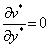(1)

Momentum Equation: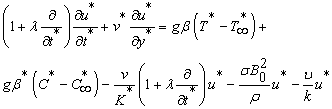(2)

Energy Equation: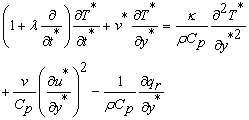(3)

Diffusion Equation: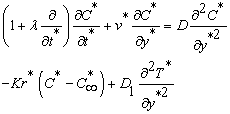(4)

In the momentum equation right hand side first two terms represent the effect of buoyancy force, third term represents the presence of non- Newtonian fluid, fourth and fifth terms represent the application of transverse magnetic field and porous medium respectively. The last two terms of RHS energy equation represent the presence of viscous dissipation and thermal radiation. Similarly the last two terms of diffusion equation represent the presence of homogeneous chemical reaction and thermal diffusion respectively.

The initial and boundary conditions are: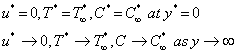(5)

The Equation (1) gives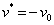(6)

Where ν0 is the constant suction velocity using the Rosseland approximation for optically thick fluids and the Taylor’s series about T neglecting the second and higher order terms we have Equation (7)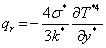(7)

Where σ*is the Stefan-bottzmann constant and is k* the mean absorption coefficient

In view of (6) and (7) the Equations (2)-(4) reduce to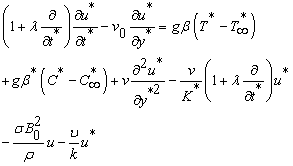(8)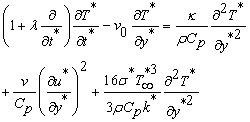(9)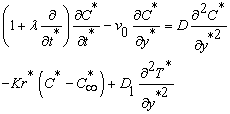(10)

On introducing the following non- dimensional quantities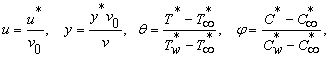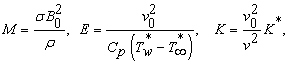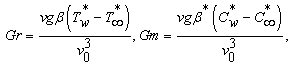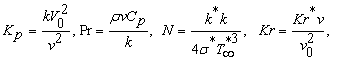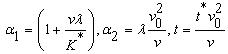(11)

In view of (11) the Equations (8)-(10), reduce to the following nondimensional form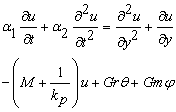(12)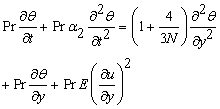(13)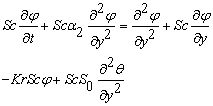(14)

Where Gr is the thermal Grashof number, Gm is the modified Grashof number, Pr is the fluid Prandle number, Sc is the Schmidt number S0 is the Soret number and Kr is the chemical reaction Parameter.

The corresponding boundary conditions reduce to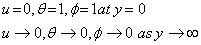(15)

#### Problem Solution

The governing Equations (12), (13) and (14) of the flow, temperature and concentration are coupled non- linear differential equations. Assuming E to be small, we write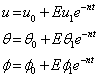(16)

Substituting Equations (16) into (12), (13) and (14) and equating the powers of E, we obtain equations to the zeroth order as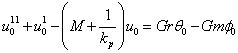(17)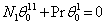(18)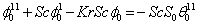(19)

The first order equations are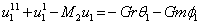(20)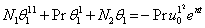(21)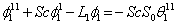(22)

The corresponding boundary conditions (15) now become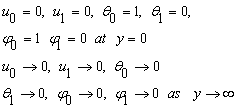(23)

Where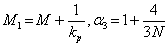Here a prime denotes the differentiation with respect to y. solving Equations (19) and (20) under the corresponding boundary conditions (23) we obtain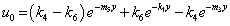(24)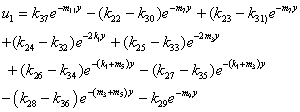(25)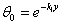(26)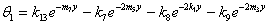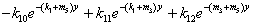(27)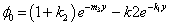(28)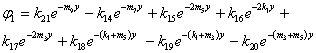(29)

Where the constants are given by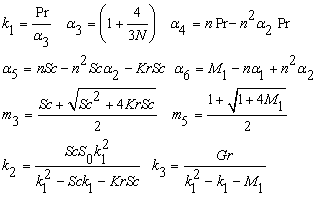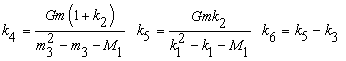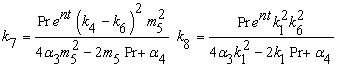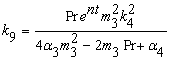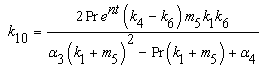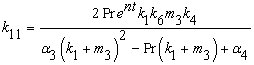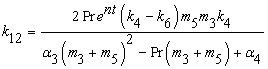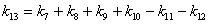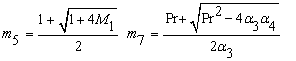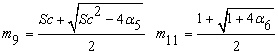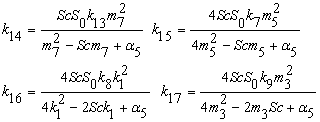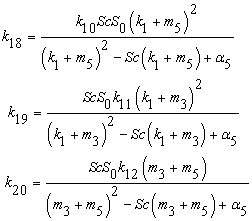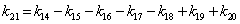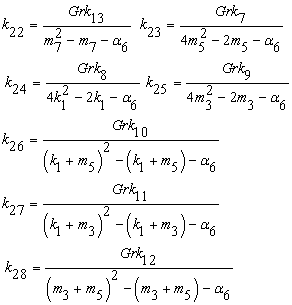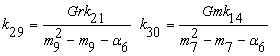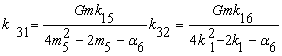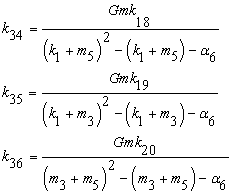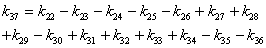#### Results and Discussion

The problem of an unsteady free convection flow of viscous, incompressible, radiating Kuvshinski fluid flow past through porous medium was formulated and solved by means perturbation method. The expressions obtained in previous section are studied with the help of figures from 1-14. The effects of various physical parameters viz., Schmidt number (Sc), thermal Grashof number (Gr), mass Grashof number (Gm), magnetic parameter (M), Prandtl number (Pr), chemical reaction parameter (Kr), thermal radiation parameter (N), Soret number (S0) are studied numerically by choosing arbitrary values. The effect of Schmidt number Sc on velocity is presented in Figure 1, as the Schmidt number increases, the velocity decreases. It is seen that velocity attains maximum near the plate and it reaches the free stream near the vicinity of the either side of the plate. This is due to the concentration buoyancy effect that decreases the fluid velocity. Figure 2 depicts the effect of Soret number on velocity field. From this figure it is observed that velocity increases with an increase in Soret number. The effect of magnetic parameter on velocity field is present in Figure 3, where velocity is observed to be decreasing with an increase in magnetic parameter. Physically this is true as an increase in magnetic field results a Lorentz’s force which has the tendency of retarding the flow. Therefore velocity obviously decreases with an increase in magnetic parameter.

In Figures 4 and 5, effects of thermal and solute buoyancy on velocity is presented, in which it is noticed that velocity increases in both the cases as both the parameters namely Grashof number and modified Grashof number increase. Effect of Prandtl number on velocity is presented in Figure 6. The numerical results show that the effect of increasing values of Prandtl number results a decrease in velocity. From Figure 7 it is observed that an increases in the thermal radiation parameter results a decrease in velocity. Effect of porosity parameter on velocity is shown in Figure 8, from this figure it is seen that velocity increases with the increasing values of porosity parameter. Chemical reaction effect on velocity is presented in Figure 9. From this numerical study it is noticed that velocity decreases with an increase in chemical reaction parameter. Effect of Schmidt number on concentration is displayed in Figure 10. From this figure it is noticed that concentration decreases with an increase in Schmidt number. Schmidt number is defined as the ratio of kinematic viscosity to the thermal diffusivity. As Schmidt number increases, usually viscosity increases and subsequently thermal diffusion decreases, therefore concentration decreases. Similar effect is noticed in the presence of chemical reaction parameter, which is shown in Figure 11 But, Figure 12 witnesses the reverse action on concentration in the presence of Soret number. In Figures 13 and 14, effect of radiation parameter and Prandtl number are presented. From these figure it is noticed that temperature decreases with the increasing in both radiation parameter and Prandtl number. Effect of Schmidt number, Soret number, magnetic number, Grashof number and modified Grashof number on Skin friction, Nusselt number and Sherwood number is presented in Table 1, from this table it is observed that, Skin friction and Nusselt number increase with an increase in Soret number, Grashof number and modified Grashof number whereas reverse phenomenon is noticed in the case of Schmidt number and magnetic parameter. But Sherwood number increases with an increase in Schmidt number, Grashof number and modified Grashof number and it decreases in the case of magnetic parameter and Soret number. Effect of chemical reaction parameter, Prandtl number, porosity parameter and radiation parameter on Skin friction, Nusselt number and Sherwood number. This table witnesses that when an increase in chemical reaction parameter results a decrease in Skin friction and Nusselt number whereas Sherwood number increases. The influence of Prandtl number decreases the shear force whereas it increases the rate of heat transfer the rate of mass transfer. The presence of porosity parameter increases the Skin friction, Nusselt number and Sherwood number. Radiation parameter increases the Nusselt number whereas it decreases the Skin friction and Sherwood number (Tables 1 and 2).

Sc S0 M Gr Gm τ Nu Sh
0.22 2 5 5 5 3.977 0.5552 0.2211
0.6 2 5 5 5 3.8308 0.5042 0.4109
0.78 2 5 5 5 3.7789 0.485 0.5001
0.96 2 5 5 5 3.7339 0.4685 0.5872
0.22 2 5 5 5 3.977 0.5552 0.2211
0.22 3 5 5 5 4.0307 0.5766 0.1627
0.22 4 5 5 5 4.0846 0.5987 0.1074
0.22 5 5 5 5 4.1387 0.6217 0.0553
0.22 2 5 5 5 3.997 0.5552 0.2211
0.22 2 6 5 5 3.7133 0.4578 0.2169
0.22 2 7 5 5 3.495 0.386 0.2139
0.22 2 8 5 5 3.3104 0.3312 0.2118
0.22 2 5 5 5 3.977 0.5552 0.2211
0.22 2 5 6 5 4.3548 0.6674 0.2259
0.22 2 5 7 5 4.7316 0.7906 0.2315
0.22 2 5 8 5 5.1074 0.9252 0.238
0.22 2 5 5 5 3.977 0.5552 0.2211
0.22 2 5 5 6 4.3901 0.6818 0.228
0.22 2 5 5 7 4.8028 0.8222 0.2366
0.22 2 5 5 8 5.2152 0.977 0.2471

Table 1: Effect of Schmidt number, Soret number, magnetic number, Grashof number and modified Grashof number on Skin friction, Nusselt number and Sherwood number.

Kr Pr Kp N τ Nu Sh
0.2 0.71 0.5 2 3.977 0.5552 0.2211
0.3 0.71 0.5 2 3.9355 0.5402 0.2791
0.4 0.71 0.5 2 3.8291 0.5274 0.5932
0.5 0.71 0.5 2 3.8701 0.517 0.3424
0.2 0.71 0.5 2 3.977 0.5552 0.2211
0.2 1 0.5 2 3.8978 1.0746 0.1518
0.2 7.1 0.5 2 3.5124 32.3181 1.5026
0.2 0.71 0.5 2 3.977 0.5552 0.2211
0.2 0.71 0.6 2 4.0779 0.5957 0.223
0.2 0.71 0.7 2 4.1546 0.6277 0.2246
0.2 0.71 0.8 2 4.215 0.6537 0.2258
0.2 0.71 0.5 2 3.977 0.5552 0.2211
0.2 0.71 0.5 3 3.9459 0.7293 0.1946
0.2 0.71 0.5 4 3.9273 0.8509 0.1784
0.2 0.71 0.5 5 3.9149 0.94 0.1673

Table 2: Effect of chemical reaction parameter, Prandtl number, porosity parameter and radiation parameter on Skin friction, Nusselt number and Sherwood number.

#### Conclusion

The problem of an unsteady free convection flow of viscous, incompressible, radiating Kuvshinski fluid flow past through porous medium was formulated and solved by means perturbation method. The following conclusions are made.

1) Velocity increases with an increase in Grashof number, modified Grashof number, Soret number, and porosity parameter but it decreases with an increase in Schmidt number, magnetic parameter, Prandtl number, radiation parameter, and chemical reaction parameter.

2) Concentration increases with an increase in Soret number but it shows reverse phenomenon in the case of Schmidt number and chemical reaction parameter.

3) Temperature decreases with an increasing in both radiation parameter and Prandtl number.

4) Skin friction increases with an increase in Soret number, Grashof number, modified Grashof number and porosity parameter, whereas it decreases in the presence of Schmidt number, magnetic parameter, chemical reaction parameter, Prandtl number and radiation parameter.

5) Nusselt number increases with an increase in Soret number, Grasof number, modified Grashof number, Prandtl number, porosity parameter and radiation parameter, where as it decreases in the presence of Schmidt number, magnetic parameter and chemical reaction parameter.

6) Sherwood number increases with an increase in Schmidt number, Grashof number, modified Grashof number, Prandtl number and porosity parameter, whereas it has different approach in the case of Soret number, magnetic parameter and radiation parameter.

#### References

Select your language of interest to view the total content in your interested language

### Article Usage

• Total views: 12659
• [From(publication date):
October-2015 - Sep 18, 2019]
• Breakdown by view type
• HTML page views : 8803

## Post your commentCan't read the image? click here to refresh
###### Peer Reviewed Journals

Make the best use of Scientific Research and information from our 700 + peer reviewed, Open Access Journals

International Conferences 2019-20

Meet Inspiring Speakers and Experts at our 3000+ Global Annual Meetings

Top